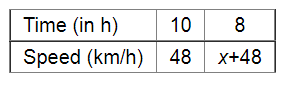# A car can finish a certain journey in 10 hours at the speed of 48 km/hr.`
Question:

A car can finish a certain journey in 10 hours at the speed of 48 km/hr. By how much should its speed be increased so that it may take only 8 hours to cover the same distance?

Solution:

Let the increased speed be x km/h.Since speed and time taken are in inverse variation, we get:

$10 \times 48=8(x+48)$

$\Rightarrow 480=8 x+384$

$\Rightarrow 8 x=480-384$

$\Rightarrow 8 x=96$

$=12$

Thus, the speed should be increased by $12 \mathrm{~km} / \mathrm{h}$.